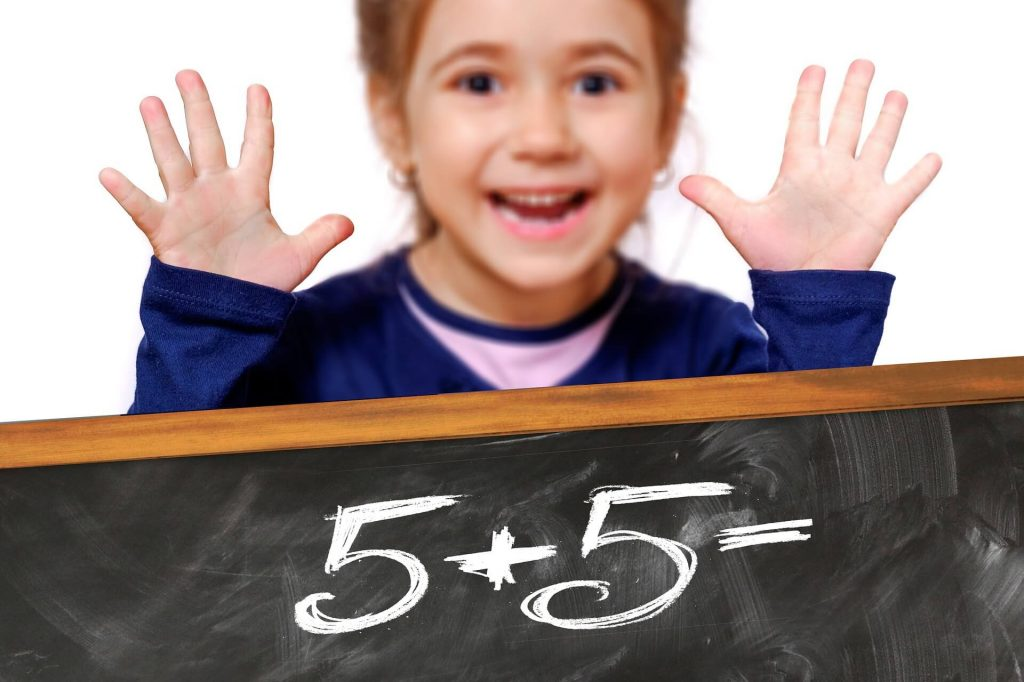By year 5, children should be confident with one- and two-step problems and using addition, subtraction, multiplication and division, and they will be able to decide on the best way of solving a problem. There is still a focus on times tables, as not all children are completely sure of these. Calculators are now being used with fractions and decimal work, and the children will use ICT to present their work. Set squares and protractors are being used for work with angles and shapes, and percentages are introduced now. Children will be practising their mental maths, as well as using written and practical methods to help them calculate. It’s also important for the children to see how their maths work links to life outside school, and to other areas of the curriculum.
Number and place value
·         Reading, writing, ordering and comparing numbers to at least 1,000,000
·         Counting forwards and backwards with positive and negative numbers
·         Rounding any number up to one million to the nearest 10, 100, 1000, 10,000, 100,000
Calculating
·         Adding and subtracting with numbers up to four digits using column addition and subtraction
·         Identifying factors and multiples of different numbers
·         Identifying prime numbers
·         Multiplying four-digit numbers with two-digit numbers using long multiplication
·         Dividing four-digit numbers by one-digit numbers using short division
·         Multiplying whole numbers and decimals by 10, 100 and 1000
·         Recognising and using square numbers and cube numbers
·         Solving problems involving all four operations

Fractions, decimals and percentages
·         Comparing and ordering fractions whose denominators are all multiples of the same number
·         Converting from mixed numbers to improper fractions
·         Adding and subtracting fractions whose denominators are multiples of the same number
·         Mutiplying proper fractions and mixed numbers by whole numbers
·         Rounding decimals with two places to the nearest whole number and to one decimal place
·         Comparing numbers with up to three decimal places
·         Begining to understand percentages
·         Knowing percentage and decimal equivalents of 1/2, 1/4, 1/5, 2/5 and 4/5

Measuring
Converting between units of measurement
Working out the perimeter and area of shapes (including irregular shapes)
Solving problems involving money and measures
Solving problems involving converting between units of time
Geometry
Drawing and measuring angles
Finding angles around a point, on a straight line and within a right angle
Statistics
Solving comparison, sum and difference problems using information presented in a line graph
Completing and interpreting information in tables, including timetables
Everyday Maths Learning @ Home
If your child has a watch, encourage them to wear it and get in the habit of looking at the time – it could be analogue or digital
Card games are perfect for playing with numbers. If you’re struggling to remember the games you played as a child, try asking the grandparents!
Most children love cooking. Following a simple recipe will give them valuable practice in measuring and weighing the ingredients and calculating cooking time. If you want to make it trickier, ask them to double or halve the quantities
Call us on please call us on 08455480140/07737413749/07943925691 to register your child for Maths intensive program